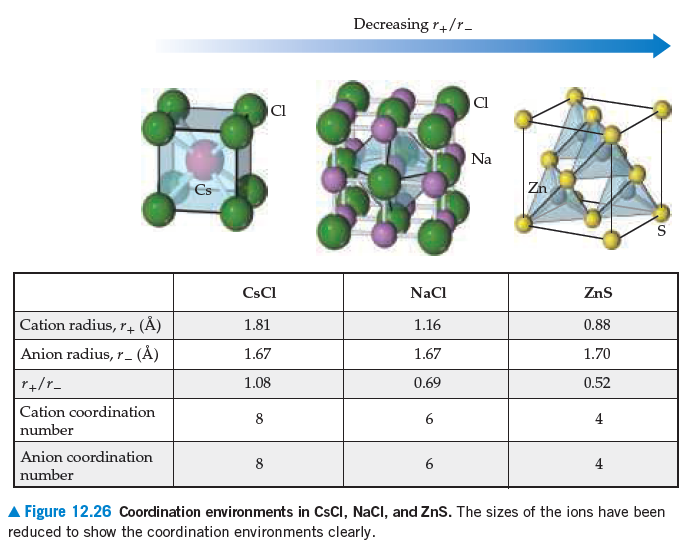# Problem: CuI, CsI, and NaI each adopt a different type of structure. The three different structures are those shown in the following figure.Use ionic radii, Na+(r = 1.61 Å) and I–(r = 2.06 Å), to predict with which structure NaI will crystallize.

###### FREE Expert Solution
96% (192 ratings)
###### Problem Details

CuI, CsI, and NaI each adopt a different type of structure. The three different structures are those shown in the following figure.Use ionic radii, Na+(r = 1.61 Å) and I(r = 2.06 Å), to predict with which structure NaI will crystallize.

Frequently Asked Questions

What scientific concept do you need to know in order to solve this problem?

Our tutors have indicated that to solve this problem you will need to apply the Unit Cell concept. You can view video lessons to learn Unit Cell. Or if you need more Unit Cell practice, you can also practice Unit Cell practice problems.